This page was auto-generated from a Jupyter notebook: CH3I_1-60eV/CH3I_1-60eV_orb30_E.

# ePSproc: CH3I wavefn test run, orb 30 ioinzation (pi anti-bonding), 1 - 60 eV¶

## Job details¶

• ePS CH3I, batch CH3I_1-60eV, orbital orb30_E

• CH3I wavefn test run, orb 30 ioinzation (pi anti-bonding), sph/ grid

• E=1:2.5:60 (24 points)

• Mon Mar 5 07:27:04 EST 2018

## Set-up¶

:

import sys
import os
import numpy as np
import epsproc as ep

from datetime import datetime as dt
timeString = dt.now()

* pyevtk not found, VTK export not available.
* plotly not found, plotly plots not available.


:

# File path only, from env var DATAFILE
# dataPath = os.getcwd()
dataFile = os.environ.get('DATAFILE', '')

:

jobInfo = ep.headerFileParse(dataFile)
molInfo = ep.molInfoParse(dataFile)

*** Job info from file header.

ePS CH3I, batch CH3I_1-60eV, orbital orb30_E
CH3I wavefn test run, orb 30 ioinzation (pi anti-bonding), sph/ grid
E=1:2.5:60 (24 points)
Mon Mar  5 07:27:04 EST 2018

*** Found orbitals
1   1 Ene =   -1177.1254 Spin =Alpha Occup =   2.000000
2   2 Ene =    -180.9096 Spin =Alpha Occup =   2.000000
3   3 Ene =    -169.6288 Spin =Alpha Occup =   2.000000
4   4 Ene =    -169.6280 Spin =Alpha Occup =   2.000000
5   5 Ene =    -169.6280 Spin =Alpha Occup =   2.000000
6   6 Ene =     -37.8993 Spin =Alpha Occup =   2.000000
7   7 Ene =     -33.0914 Spin =Alpha Occup =   2.000000
8   8 Ene =     -33.0881 Spin =Alpha Occup =   2.000000
9   9 Ene =     -33.0881 Spin =Alpha Occup =   2.000000
10  10 Ene =     -24.2551 Spin =Alpha Occup =   2.000000
11  11 Ene =     -24.2539 Spin =Alpha Occup =   2.000000
12  12 Ene =     -24.2539 Spin =Alpha Occup =   2.000000
13  13 Ene =     -24.2506 Spin =Alpha Occup =   2.000000
14  14 Ene =     -24.2506 Spin =Alpha Occup =   2.000000
15  15 Ene =     -11.2780 Spin =Alpha Occup =   2.000000
16  16 Ene =      -7.2111 Spin =Alpha Occup =   2.000000
17  17 Ene =      -5.4461 Spin =Alpha Occup =   2.000000
18  18 Ene =      -5.4388 Spin =Alpha Occup =   2.000000
19  19 Ene =      -5.4388 Spin =Alpha Occup =   2.000000
20  20 Ene =      -2.3765 Spin =Alpha Occup =   2.000000
21  21 Ene =      -2.3724 Spin =Alpha Occup =   2.000000
22  22 Ene =      -2.3724 Spin =Alpha Occup =   2.000000
23  23 Ene =      -2.3631 Spin =Alpha Occup =   2.000000
24  24 Ene =      -2.3631 Spin =Alpha Occup =   2.000000
25  25 Ene =      -0.9966 Spin =Alpha Occup =   2.000000
26  26 Ene =      -0.7971 Spin =Alpha Occup =   2.000000
27  27 Ene =      -0.6101 Spin =Alpha Occup =   2.000000
28  28 Ene =      -0.6101 Spin =Alpha Occup =   2.000000
29  29 Ene =      -0.4641 Spin =Alpha Occup =   2.000000
30  30 Ene =      -0.3629 Spin =Alpha Occup =   2.000000
31  31 Ene =      -0.3629 Spin =Alpha Occup =   2.000000

*** Found atoms
Z = 53 ZS = 53 r =   0.0000000000   0.0000000000   1.9127440000
Z =  6 ZS =  6 r =   0.0000000000   0.0000000000  -0.2324950000
Z =  1 ZS =  1 r =  -0.5117920000   0.8864500000  -0.5600500000
Z =  1 ZS =  1 r =  -0.5117920000  -0.8864500000  -0.5600500000
Z =  1 ZS =  1 r =   1.0235850000   0.0000000000  -0.5600500000

:

# Scan file(s) for various data types...

# For dir scan
# dataXS = ep.readMatEle(fileBase = dataPath, recordType = 'CrossSection')
# dataMatE = ep.readMatEle(fileBase = dataPath, recordType = 'DumpIdy')

# For single file
dataXS = ep.readMatEle(fileIn = dataFile, recordType = 'CrossSection')
dataMatE = ep.readMatEle(fileIn = dataFile, recordType = 'DumpIdy')


*** ePSproc readMatEle(): scanning files for CrossSection segments.

*** Scanning file(s)
['/home/paul/ePS_results/CH3I/CH3I_1-60eV/CH3I_1-60eV_orb30_E.inp.out']

*** Reading ePS output file:  /home/paul/ePS_results/CH3I/CH3I_1-60eV/CH3I_1-60eV_orb30_E.inp.out
Expecting 4 symmetries.
Scanning CrossSection segments.
Expecting 5 CrossSection segments.
Found 5 CrossSection segments (sets of results).
Processed 5 sets of CrossSection file segments, (0 blank)
*** ePSproc readMatEle(): scanning files for DumpIdy segments.

*** Scanning file(s)
['/home/paul/ePS_results/CH3I/CH3I_1-60eV/CH3I_1-60eV_orb30_E.inp.out']

*** Reading ePS output file:  /home/paul/ePS_results/CH3I/CH3I_1-60eV/CH3I_1-60eV_orb30_E.inp.out
Expecting 4 symmetries.
Scanning CrossSection segments.
Expecting 96 DumpIdy segments.
Found 96 dumpIdy segments (sets of matrix elements).

Processing segments to Xarrays...
Processed 96 sets of DumpIdy file segments, (0 blank)


## Job & molecule info¶

:

ep.jobSummary(jobInfo, molInfo);


*** Job summary data
ePS CH3I, batch CH3I_1-60eV, orbital orb30_E
CH3I wavefn test run, orb 30 ioinzation (pi anti-bonding), sph/ grid
E=1:2.5:60 (24 points)
Mon Mar  5 07:27:04 EST 2018

Electronic structure input: '/media/hockettp/StoreM/ePolyScat_stuff/CH3I/electronic_structure/CH3I_ref_opt_SPK-AQZP_C3v.molden'
Initial state occ:               [2 2 2 4 2 2 4 2 4 4 2 2 2 4 2 4 4 2 2 4 2 4]
Final state occ:                 [2 2 2 4 2 2 4 2 4 4 2 2 2 4 2 4 4 2 2 4 2 3]
IPot (input vertical IP, eV):            9.54

*** Additional orbital info (SymProd)
Ionizing orb:                    [0 0 0 0 0 0 0 0 0 0 0 0 0 0 0 0 0 0 0 0 0 1]
Ionizing orb sym:                ['E']
Orb energy (eV):                 [-9.87501199]
Orb energy (H):                  [-0.3629]
Orb energy (cm^-1):              [-79647.34372628]
Threshold wavelength (nm):       125.5534652149492

*** Warning: some orbital convergences outside single-center expansion convergence tolerance (0.01):
[[1.0000000e+00 2.0136460e-02]
[2.0000000e+00 5.8847706e-01]
[3.0000000e+00 3.3933218e-01]
[4.0000000e+00 9.3922660e-02]
[5.0000000e+00 9.3922660e-02]
[6.0000000e+00 7.6662327e-01]
[7.0000000e+00 8.6152457e-01]
[8.0000000e+00 7.6041242e-01]
[9.0000000e+00 7.6041242e-01]
[1.0000000e+01 6.8940314e-01]
[1.1000000e+01 5.8978015e-01]
[1.2000000e+01 5.8978015e-01]
[1.3000000e+01 3.8623257e-01]
[1.4000000e+01 3.8623257e-01]
[1.6000000e+01 9.4364590e-01]
[1.7000000e+01 9.6540337e-01]
[1.8000000e+01 9.2145796e-01]
[1.9000000e+01 9.2145796e-01]
[2.0000000e+01 9.5859657e-01]
[2.1000000e+01 9.3960899e-01]
[2.2000000e+01 9.3960899e-01]
[2.3000000e+01 9.1904483e-01]
[2.4000000e+01 9.1904483e-01]]

*** Molecular structure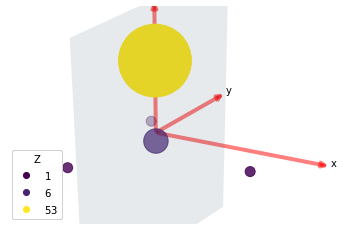## 1-photon ePS Cross-Sections¶

Plot 1-photon cross-sections and $$beta_2$$ parameters (for an unaligned ensemble) from ePS calculations. These are taken directly from the ePS output file, CrossSection segments. See the ePS manual, GetCro command, for further details <https://www.chem.tamu.edu/rgroup/lucchese/ePolyScat.E3.manual/GetCro.html>__.

### Cross-sections by symmetry & type¶

Types correspond to:

• ‘L’: length gauge results.

• ‘V’: velocity gauge results.

• ‘M’: mixed gauge results.

Symmetries correspond to allowed ionizing transitions for the molecular point group (IRs typically corresponding to (x,y,z) polarization geometries), see the ePS manual for a list of symmetries. Symmetry All corresponds to the sum over all allowed sets of symmetries.

Cross-section units are MBarn.

:

# Plot cross sections using Xarray functionality
# Set here to plot per file - should add some logic to combine files.
for data in dataXS:
daPlot = data.sel(XC='SIGMA')
daPlot.plot.line(x='Eke', col='Type')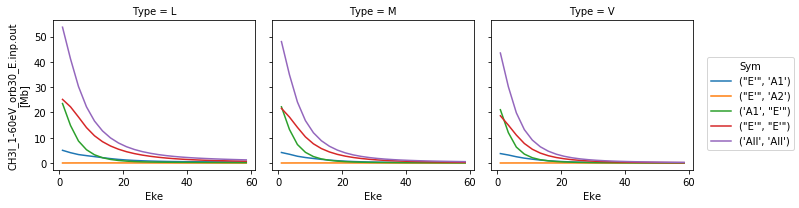### $$\beta_{2}$$ by symmetry & type¶

Types & symmetries as per cross-sections. Normalized $$\beta_{2}$$ paramters, dimensionless.

:

# Repeat for betas
for data in dataXS:
daPlot = data.sel(XC='BETA')
daPlot.plot.line(x='Eke', col='Type')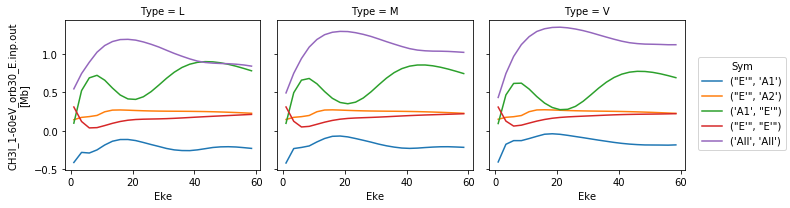## Dipole matrix elements¶

For 1-photon ionization. These are taken directly from ePS DumpIdy segments. See the ePS manual, DumpIdy command, for further details <https://www.chem.tamu.edu/rgroup/lucchese/ePolyScat.E3.manual/DumpIdy.html>__.

:

# Set threshold for significance, only matrix elements with abs values > thres % will be plotted
thres = 0.1

:

# Plot for each fie
for data in dataMatE:
# Plot with sensible defaults - all dims with lmPlot()

# Plot only values > theshold
daPlot, daPlotpd, legendList, gFig = ep.lmPlot(data, thres = thres, thresType = 'pc', figsize = (15,10))

# Plot phases, with unwrap
daPlot, daPlotpd, legendList, gFig = ep.lmPlot(data, thres = thres, thresType = 'pc', figsize = (15,10), pType='phaseUW')

/home/paul/anaconda3/envs/ePSproc-v1.2/lib/python3.7/site-packages/xarray/core/nputils.py:223: RuntimeWarning: All-NaN slice encountered
result = getattr(npmodule, name)(values, axis=axis, **kwargs)

Plotting data CH3I_1-60eV_orb30_E.inp.out, pType=a, thres=1.419151168894083, with Seaborn

/home/paul/anaconda3/envs/ePSproc-v1.2/lib/python3.7/site-packages/xarray/core/nputils.py:223: RuntimeWarning: All-NaN slice encountered
result = getattr(npmodule, name)(values, axis=axis, **kwargs)
/home/paul/anaconda3/envs/ePSproc-v1.2/lib/python3.7/site-packages/numpy/lib/function_base.py:1520: RuntimeWarning: invalid value encountered in greater
_nx.copyto(ddmod, pi, where=(ddmod == -pi) & (dd > 0))
/home/paul/anaconda3/envs/ePSproc-v1.2/lib/python3.7/site-packages/numpy/lib/function_base.py:1522: RuntimeWarning: invalid value encountered in less
_nx.copyto(ph_correct, 0, where=abs(dd) < discont)

Plotting data CH3I_1-60eV_orb30_E.inp.out, pType=phaseUW, thres=1.419151168894083, with Seaborn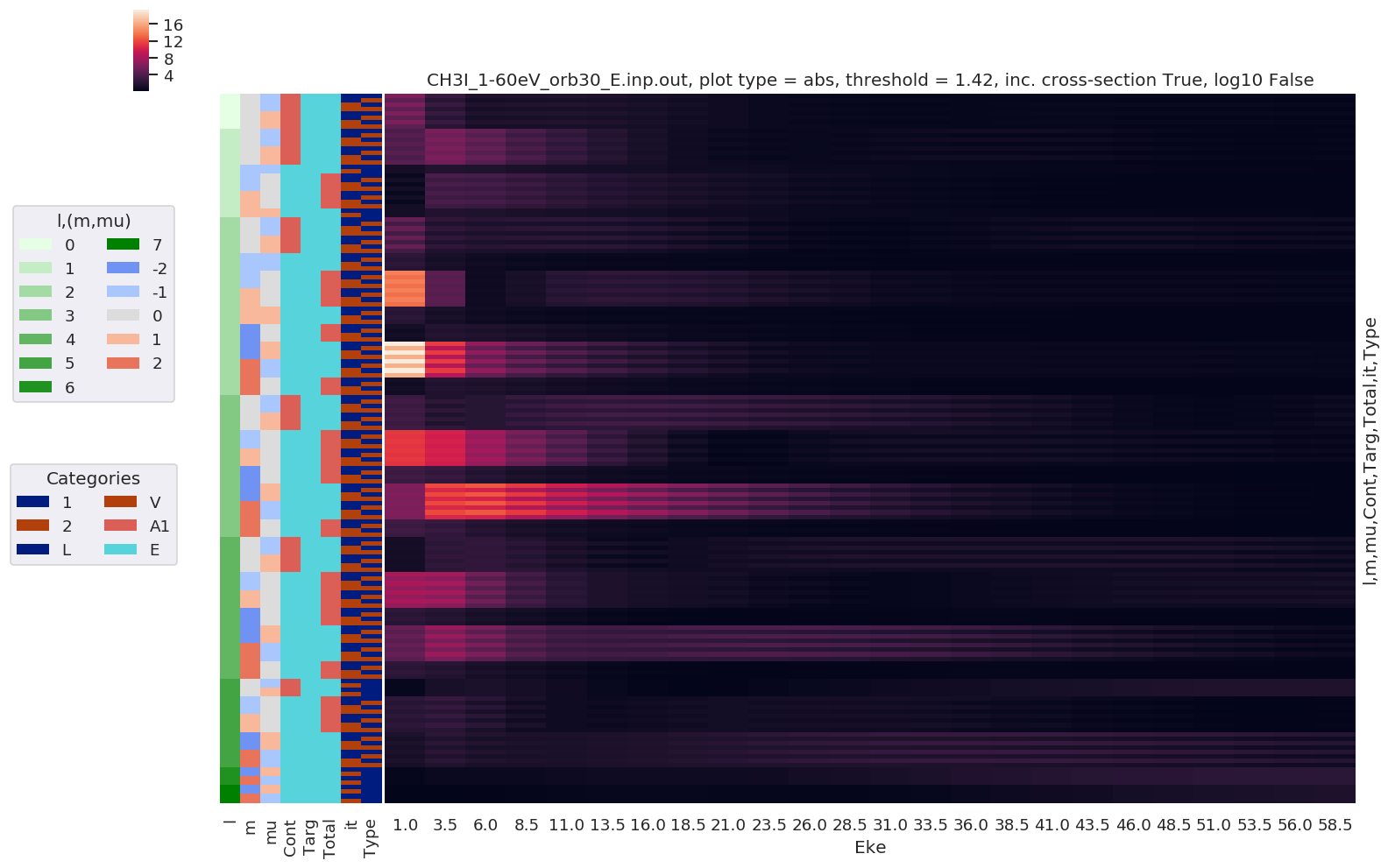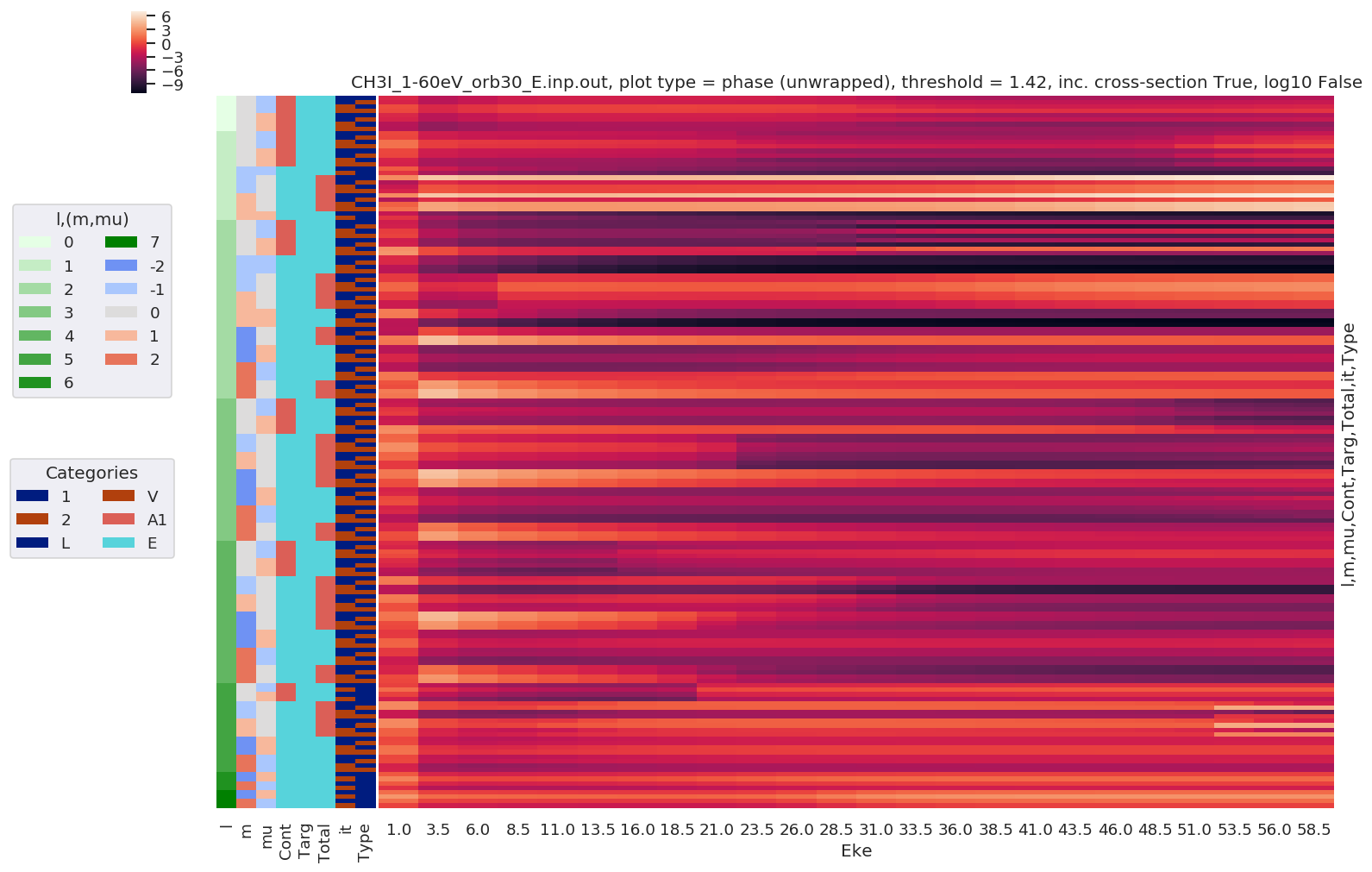Calculated MF $$\beta$$ parameters, using ePS dipole matrix elements. These are calculated by ep.mfblm(), as a function of energy and polarization geometry. See the ePSproc docs on ep.mfblm() <https://epsproc.readthedocs.io/en/latest/modules/epsproc.MFBLM.html>__ for further details, and this demo notebook.

:

# Set pol geoms - these correspond to (z,x,y) in molecular frame (relative to principle/symmetry axis)
eAngs = ep.setPolGeoms()

:

# Drop threshold for MF calcs
thres = 1e-3
# Calculate for each fie & pol geom
# TODO - file logic, and parallelize
BLM = []
for data in dataMatE:
BLM.append(ep.mfblmEuler(data, selDims = {'Type':'L'}, eAngs = eAngs, thres = thres,
SFflag = True, verbose = 0))  # Run for all Eke, selected gauge only

:

# Save BLM data - defaults to working dir and 'ep_timestamp' file
# TODO - testing for array/multiple file case
for data in BLM:
fileName = dataFile + '_BLM-L_' + timeString.strftime('%Y-%m-%d_%H-%M-%S')
ep.writeXarray(data, fileName = fileName)

['Written to h5netcdf format', '/home/paul/ePS_results/CH3I/CH3I_1-60eV/CH3I_1-60eV_orb30_E.inp.out_BLM-L_2020-02-10_09-08-11.nc']

/home/paul/anaconda3/envs/ePSproc-v1.2/lib/python3.7/site-packages/h5netcdf/core.py:481: H5pyDeprecationWarning: other_ds.dims.create_scale(ds, name) is deprecated. Use ds.make_scale(name) instead.
h5ds.dims.create_scale(h5ds, scale_name)

:

# Normalize and plot results
for BLMplot in BLM:
# Plot unnormalized B00 only, real part
# This is/should be in units of MBarn (TBC).
#     BLMplot.where(np.abs(BLMplot) > thres, drop = True).real.squeeze().sel({'l':0, 'm':0}).plot.line(x='Eke', col='Euler');
BLMplot.XS.real.squeeze().plot.line(x='Eke', col='Euler');

# Plot values normalised by B00 - now set in calculation function
# Plot results with lmPlot(), ordering by Euler sets
# Version with (semi-manual) Euler grouping
daPlot, daPlotpd, legendList, gFig = ep.lmPlot(BLMplot.swap_dims({'Euler':'Labels'}), SFflag = False, eulerGroup = True,
thresType = 'pc', thres = thres,
plotDims = ('Labels','l','m'),
figsize = (15,10))

Plotting data CH3I_1-60eV_orb30_E.inp.out, pType=a, thres=0.001017613059022104, with Seaborn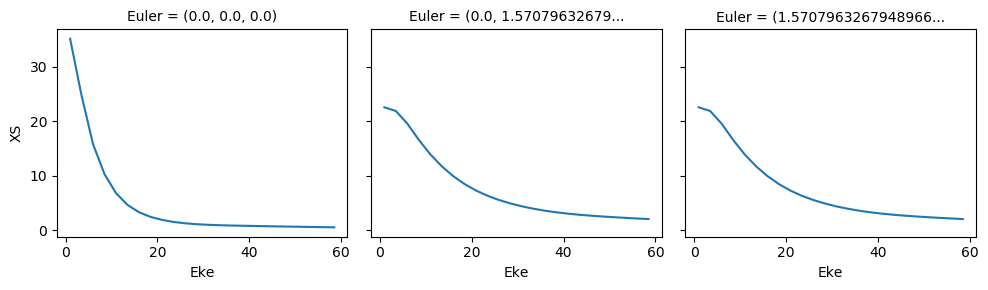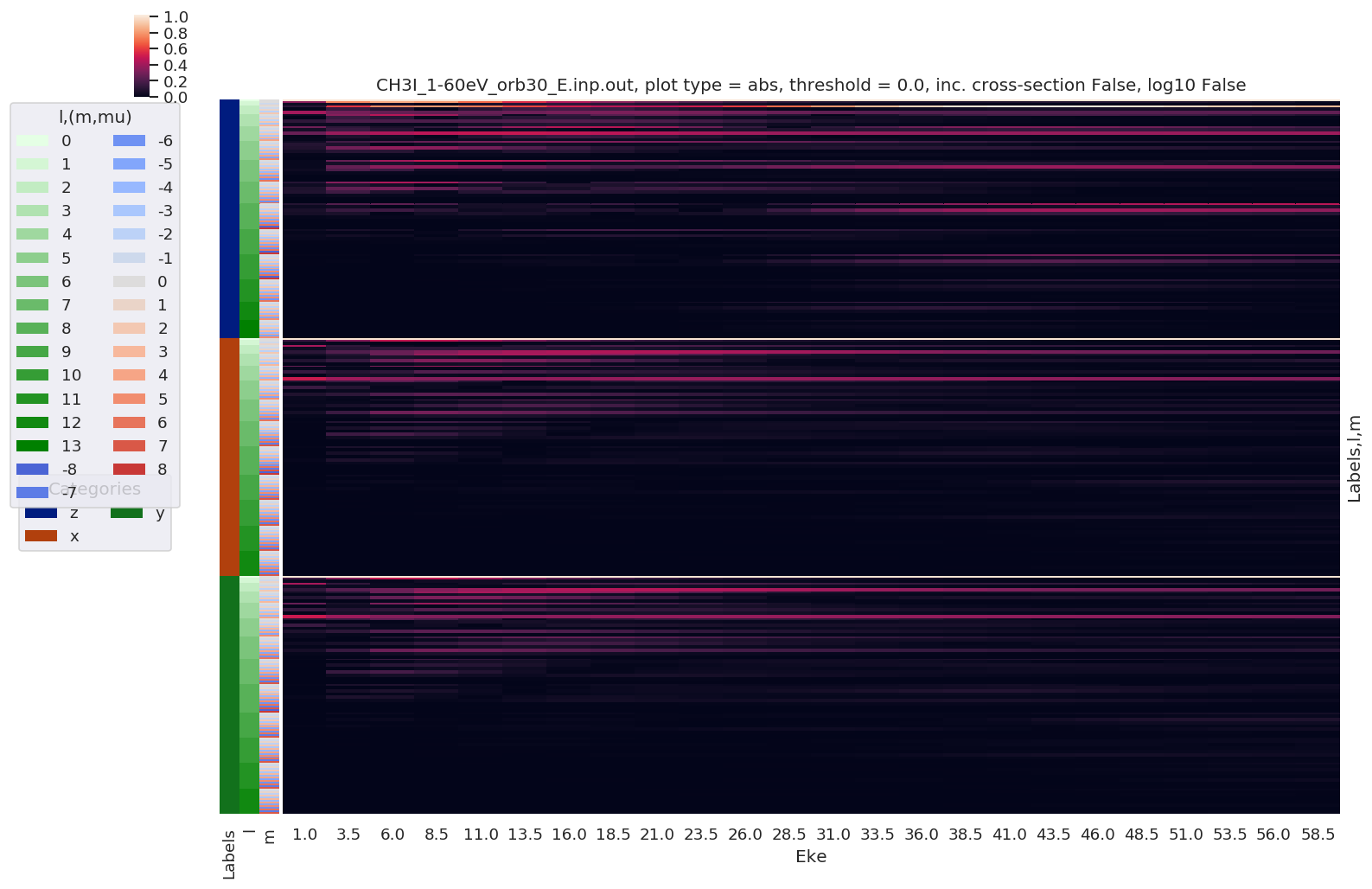## Error & consistency checks¶

:

# Check SF values
for data in dataMatE:
# Plot values, single plot
data.SF.pipe(np.abs).plot.line(x='Eke')
data.SF.real.plot.line(x='Eke')
data.SF.imag.plot.line(x='Eke')

# Plot values, facet plot
#     data.SF.pipe(np.abs).plot.line(x='Eke', col='Sym')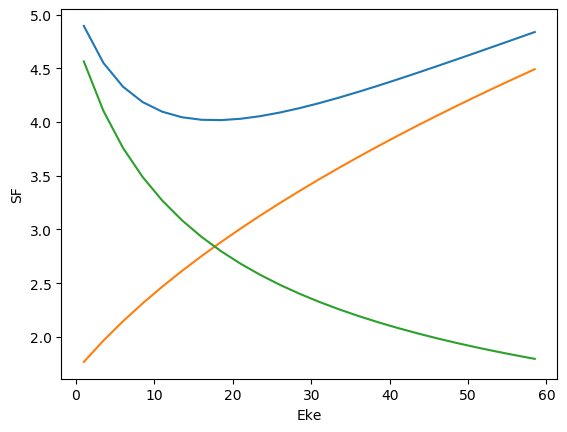:

# Compare calculated BLMs for L and V types (dafault above for L)

# Calculate for each fie & pol geom, and compare.
BLMv = []
BLMdiff = []
for n, data in enumerate(dataMatE):
BLMv.append(ep.mfblmEuler(data, selDims = {'Type':'V'}, eAngs = eAngs, thres = thres,
SFflag = True, verbose = 0))  # Run for all Eke, selected gauge only

BLMdiff.append(BLM[n] - BLMv[n])
BLMdiff[n]['dXS'] = BLM[n].XS - BLMv[n].XS  # Set XS too, dropped in calc above

BLMdiff[n].attrs['dataType'] = 'matE'

:

# Save BLM data - defaults to working dir and 'ep_timestamp' file
# TODO - testing for array/multiple file case
for data in BLMv:
fileName = dataFile + '_BLM-V_' + timeString.strftime('%Y-%m-%d_%H-%M-%S')
ep.writeXarray(data, fileName = fileName)

['Written to h5netcdf format', '/home/paul/ePS_results/CH3I/CH3I_1-60eV/CH3I_1-60eV_orb30_E.inp.out_BLM-V_2020-02-10_09-08-11.nc']

/home/paul/anaconda3/envs/ePSproc-v1.2/lib/python3.7/site-packages/h5netcdf/core.py:481: H5pyDeprecationWarning: other_ds.dims.create_scale(ds, name) is deprecated. Use ds.make_scale(name) instead.
h5ds.dims.create_scale(h5ds, scale_name)

:

# Normalize and plot results
for BLMplot in BLMv:
# Plot unnormalized B00 only, real part
# This is/should be in units of MBarn (TBC).
#     BLMplot.where(np.abs(BLMplot) > thres, drop = True).real.squeeze().sel({'l':0, 'm':0}).plot.line(x='Eke', col='Euler');
BLMplot.XS.real.squeeze().plot.line(x='Eke', col='Euler');

# Plot values normalised by B00 - now set in calculation function
# Plot results with lmPlot(), ordering by Euler sets
# Version with (semi-manual) Euler grouping
daPlot, daPlotpd, legendList, gFig = ep.lmPlot(BLMplot.swap_dims({'Euler':'Labels'}), SFflag = False, eulerGroup = True,
thresType = 'pc', thres = thres,
plotDims = ('Labels','l','m'),
figsize = (15,10))

Plotting data CH3I_1-60eV_orb30_E.inp.out, pType=a, thres=0.001, with Seaborn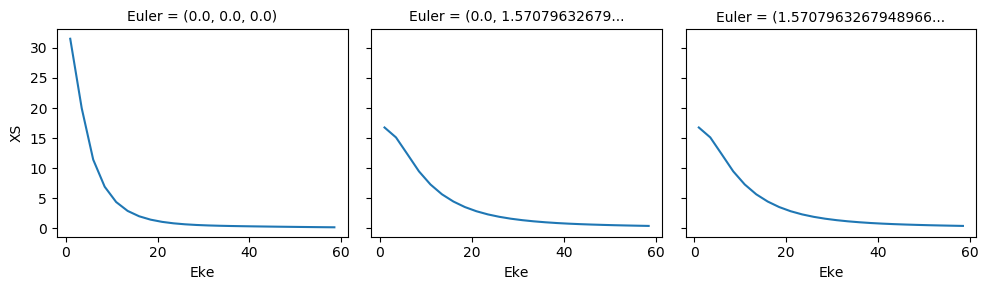:

# Difference between 'L' and 'V' results
# NOTE - this currently drops XS

print('Differences, L vs. V gauge BLMs')

for BLMplot in BLMdiff:
maxDiff = BLMplot.max()
print(f'Max difference in BLMs (L-V): {0}', maxDiff.data)

if np.abs(maxDiff) > thres:
# Plot B00 only, real part
#         BLMplot.where(np.abs(BLMplot) > thres, drop = True).real.squeeze().sel({'l':0, 'm':0}).plot.line(x='Eke', col='Euler');
BLMplot.dXS.real.squeeze().plot.line(x='Eke', col='Euler');

# Plot values normalised by B00 - now set in calculation function
# Plot results with lmPlot(), ordering by Euler sets
# Version with (semi-manual) Euler grouping
daPlot, daPlotpd, legendList, gFig = ep.lmPlot(BLMplot.swap_dims({'Euler':'Labels'}), SFflag = False, eulerGroup = True,
thresType = 'pc', thres = thres,
plotDims = ('Labels','l','m'),
figsize = (15,10))


Differences, L vs. V gauge BLMs
Max difference in BLMs (L-V): 0 (0.332299981441156+2.9950448929007464e-17j)
Plotting data (No filename), pType=a, thres=0.00033229998144115603, with Seaborn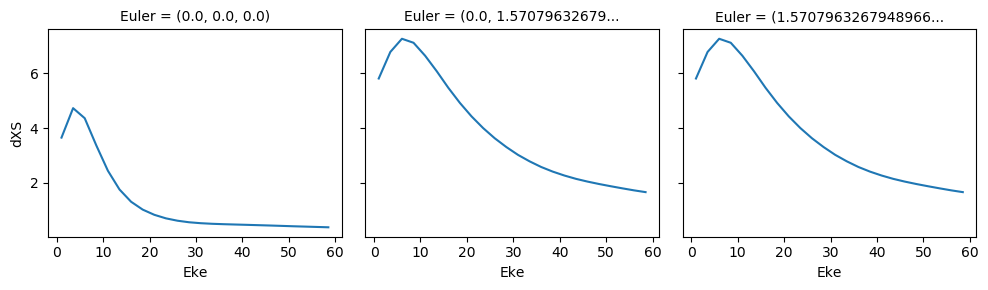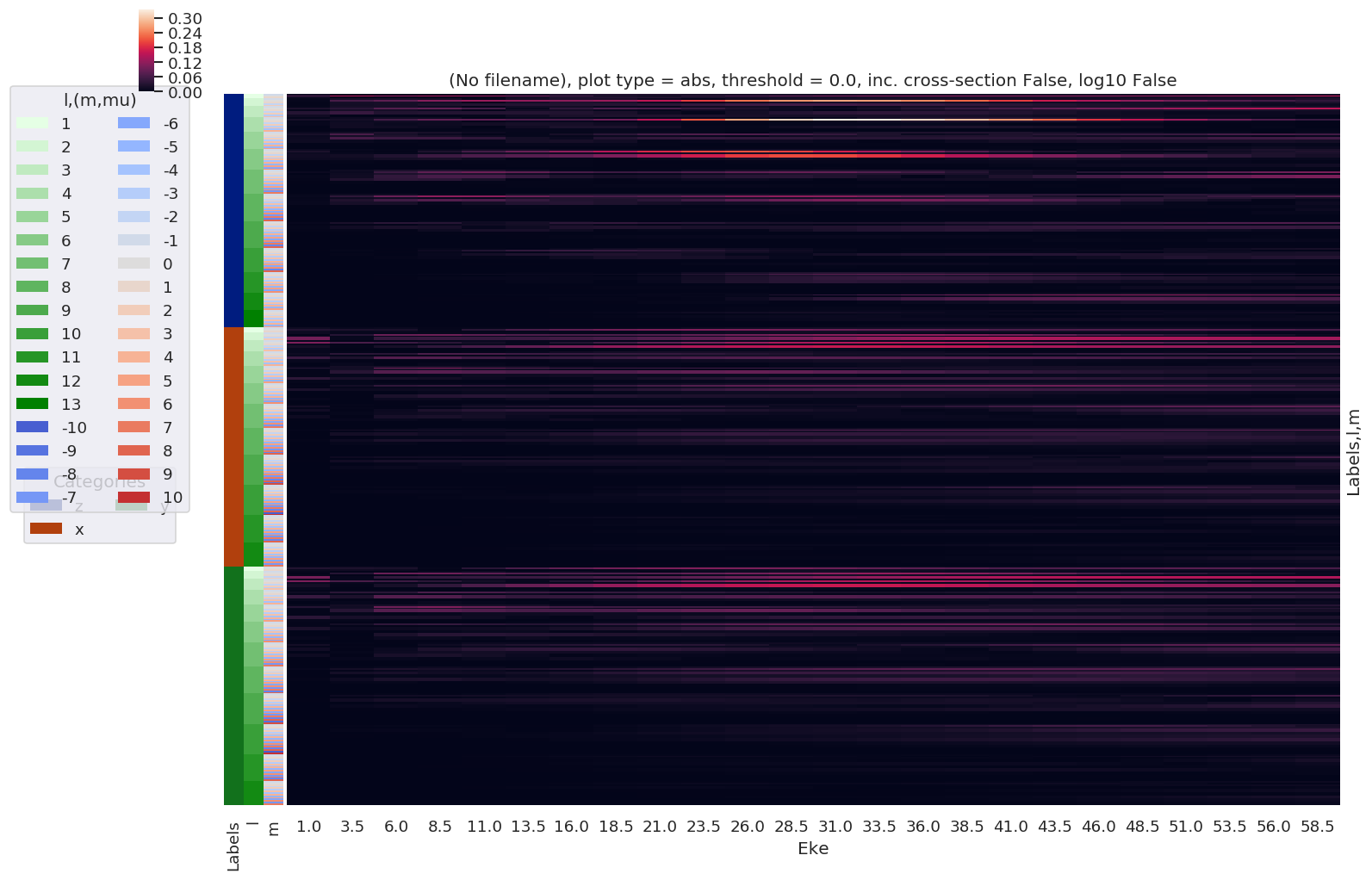:

# Check imaginary components - should be around machine tolerance.
print('Machine tolerance: ', np.finfo(float).eps)
for BLMplot in BLM:
maxImag = BLMplot.imag.max()
print(f'Max imaginary value: {0}', maxImag.data)

#     BLMplot.where(np.abs(BLMplot) > thres, drop = True).imag.squeeze().plot.line(x='Eke', col='Euler');

BLMplot = ep.matEleSelector(BLMplot, thres=thres, dims = 'Eke')
BLMplot.imag.squeeze().plot.line(x='Eke', col='Euler');

Machine tolerance:  2.220446049250313e-16
Max imaginary value: 0 0.5111423032385483## Version info¶

### Original job details¶

:

print(jobInfo['ePolyScat'])
print('Run: ' + jobInfo['Starting'].split('at'))

ePolyScat Version E3
Run:  2018-03-13  03:21:35.541 (GMT -0400)


### ePSproc details¶

:

templateVersion = '0.0.6'
templateDate = '12/01/20'

:

%load_ext version_information

:

%version_information epsproc, xarray

:

SoftwareVersion
Python3.7.5 64bit [GCC 7.3.0]
IPython7.9.0
OSLinux 5.0.0 36 generic x86_64 with debian buster sid
epsproc1.2.4
xarray0.14.0
Tue Feb 11 13:31:20 2020 EST
:

print('Run: {}'.format(timeString.strftime('%Y-%m-%d_%H-%M-%S')))
host = !hostname
print('Host: {}'.format(host))

Run: 2020-02-10_09-08-11
Host: jake


## Cite this dataset¶

Hockett, Paul (2018). ePSproc: CH3I wavefn test run, orb 30 ioinzation (pi anti-bonding), 1 - 60 eV. Dataset on Zenodo. DOI: 10.5281/zenodo.3660716. URL: https://phockett.github.io/ePSdata/CH3I_1-60eV/CH3I_1-60eV_orb30_E.html

Bibtex:

@data{CH3I wavefn test run, orb 30 ioinzation (pi anti-bonding), 1 - 60 eV,
title = {ePSproc: CH3I wavefn test run, orb 30 ioinzation (pi anti-bonding), 1 - 60 eV}
author = {Hockett, Paul},
doi = {10.5281/zenodo.3660716},
publisher = {Zenodo},
year = {2018},
url = {https://phockett.github.io/ePSdata/CH3I_1-60eV/CH3I_1-60eV_orb30_E.html}
}


See citation notes on ePSdata for further details.